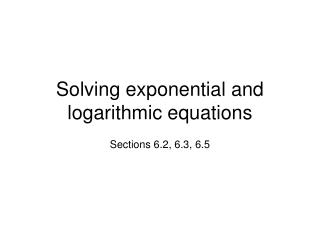DownloadDownload PresentationSolving exponential and logarithmic equations

# Solving exponential and logarithmic equations

Download Presentation## Solving exponential and logarithmic equations

- - - - - - - - - - - - - - - - - - - - - - - - - - - E N D - - - - - - - - - - - - - - - - - - - - - - - - - - -
##### Presentation Transcript

1. Solving exponential and logarithmic equations Sections 6.2, 6.3, 6.5

2. The details about functions • If a relationship is a function, then for every x value, there is only one y value. Another way to put this is, if for some numbers u and v, then . Solve 5 = x – 2. We do it by applying the function f(x) = x + 2. Because of the above statement, we can apply the function to both sides (getting x = 7) and the equality holds. We’ll do the same with logarithmic and exponential functions.

3. The details about one-to-one • If a function is one-to-one, then for every y value, there is only one x value. So if for some numbers u and v, then . Solve . Since is one-to-one, then we can say x = 4. Solve . Since is not one-to-one, then we cannot say x = 4. What else could x be?

4. The logarithmic and exponential relationships are both functions and 1-1.

5. These rules will help us solve equations. Rules • 1. Since is 1-1, we can say that if , then • . (Here u and v are real numbers, and a is a positive real number, not 1.) • 2. Since is 1-1, we can say that if , then . (Here M, N, and a are positive real numbers, and a is not 1.) If then If then

6. These rules will help us solve equations. Rules • 3. Since is a function, we can say that if , then . (Here u and v are real numbers, and a is a positive real number, not 1.) • 4. Since is a function, we can say that if , then . (Here M, N, and a are positive real numbers, and a is not 1.)

7. Remember and undo each other. They are inverses and so whatever the one does, the other will undo. The four rules are connected by this inverse relationship. • We’re solving equations in these sections. Remember we are trying to find the x that makes the equation true. We will need to keep a lot in our minds. • 1.) the four rules just discussed • 2.) the fact that and are inverses • 3.) the fact that and are equivalent • 4.) the logarithm rules from 6.4

8. expl: • Solve • Two different ways: • Method 1: Use equivalency of and …and simplify.

9. Use the inverse relationship between and . • Method 2: Rule 3 Apply to both sides. The logarithm function undid the exponential function and left 4w on the left.

10. Use equivalency of and expl: • Solve • Three different ways: • Method 1: Use the Change of Base formula on left …and simplify. Check

11. Rule 4 “take log of both sides” • Method 2: …and simplify.

12. Rule 4 “take natural log of both sides” • Method 3: …and simplify. Unbury the t.

13. expl: #7, pg 471 • Solve • Method 1: Use equivalency of and Check

14. The plan is to combine the logs so deal with the 3 on the first term. • Method 2: Use equivalency of and … and unbury the x.

15. expl: #10, pg 471 Use the rules from 6.4 to simplify the left side. • Solve Use equivalency of and The quadratic formula gets us x is -1 or 4. We check both to see that -1 does not work so 4 is our only solution.

16. expl: #89, pg 455 Use equivalency of and • Solve The base b must be positive and not 1. Check: does not exist. So -2 is not a solution. The solution 2 does work.

17. Write both sides as 5 raised to some power. Then we can say the exponents are equal. expl: 44, pg 442 • Solve Check:

18. expl: #48, pg 471 • Solve graphically. • Using the change of base formula for the left side, we will graph • and see where they intersect.

19. The solution to the equation is the x value where the two sides intersect.

20. Worksheet • “Using log rules to solve equations” goes through problems step-by-step. We look at both algebraic and graphical solutions. • Comments on worksheet: • 1.) Re #3: Do not rewrite as • It will not graph the correct function. • 2.) Re #4: Here we want the left side graphed as • , not as

21. Worksheet • “Logarithmic and exponential applications: Intensity of sound” introduces the loudness of sounds, measured in decibels, as a neat little example of the logarithmic function. We practice solving equations using this application. • 6.2 homework: 43, 50, 52 • 6.3 homework: 85, 87, 91, 101, 111, 115, 122 • 6.5 homework: 1, 5, 9, 15, 17, 29, 37, 47, 49